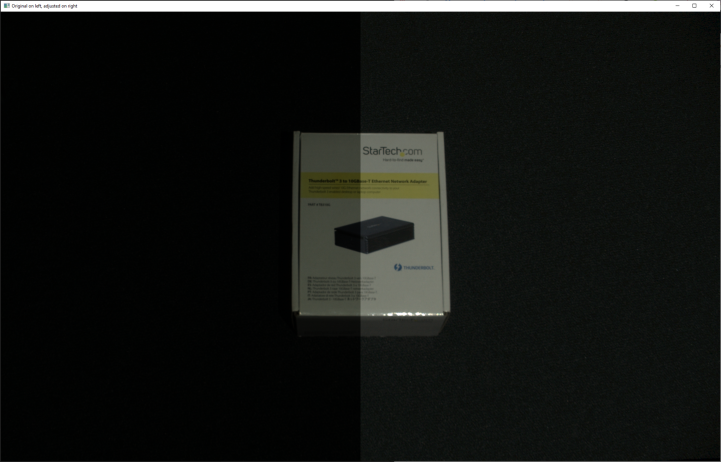# Gamma Correction

This tutorial demonstrates how to capture a 2D image with a configurable gamma correction.

First, we connect to the camera.

```camera = app.connect_camera()
```

Then we get the gamma as command line argument from user.

```user_options = _options()
print(f"Capturing with gamma correction: {user_options.gamma}")
```

We then capture the image with no gamma correction (gamma = 1.0) and with given gamma correction (in this case, gamma = 0.6).

```bgr_original = _capture_bgr_image(camera, 1.0)
```

The complete implementation of the function to capture a color image is given below.

```def _capture_bgr_image(camera, gamma):
"""Capture and extract 2D image, then convert from RGBA and return BGR.

Args:
camera: Zivid Camera handle
gamma: Gamma correction value

Returns:
BGR image (HxWx3 darray)

"""
print("Configuring Settings")
settings_2d = zivid.Settings2D(
acquisitions=[zivid.Settings2D.Acquisition()],
)
settings_2d.processing.color.gamma = gamma

print("Capturing 2D frame")
with camera.capture(settings_2d) as frame_2d:
image = frame_2d.image_rgba()
rgba = image.copy_data()
bgr = cv2.cvtColor(rgba, cv2.COLOR_RGBA2BGR)
return bgr

```

Lastly, we display both images.

```width = (int)(bgr_original.shape / 2)
combined_image = np.hstack([bgr_original[:, :width], bgr_adjusted[:, -width:]])
cv2.imshow("Original on left, adjusted on right", combined_image)
print("Press any key to continue")
cv2.waitKey(0)
```